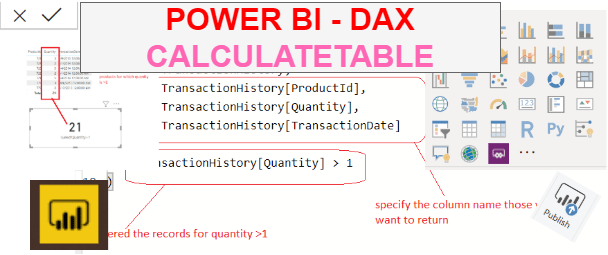Home » dax calculate

# dax calculate## CALCULATETABLE function DAX

CALCULATETABLE function is a power bi filter function in DAX that evaluates a table expression in a context modified by the given filters. It returns a table of values.

DAX SYNTAX

CALCULATETABLE(,,,…)

18,175 total views,  5 views today